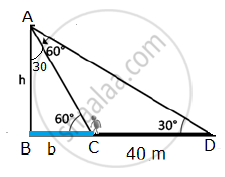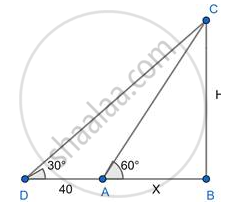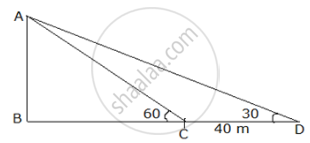# A person standing on the bank of river observes that the angle of elevation of the top of a tree standing on the opposite bank is 60° - Geometry

Sum

A person standing on the bank of river observes that the angle of elevation of the top of a tree standing on the opposite bank is 60°. When he moves 40 m away from the bank, he finds the angle of elevation to be 30°. Find the height of the tree and width of the river. (sqrt 3=1.73)

#### Solution 1

Let AB = height of the tower = h metres
In the right angled ΔABC and right angled ΔABD,tan60^@=h/(BC)=sqrt(3) rArr BC=h/sqrt(3)

tan30^@=h/(BD)=1/sqrt(3) rArr BD=hsqrt(3)

Now, BD – BC = 40

hsqrt(3)-h/sqrt(3)=40

(3h-h)/sqrt3=40

2h=40sqrt3

h=20sqrt3 m

In right angled ΔABC,

tan30°=1/sqrt3

(BC)/h=1/sqrt3

(BC)/(20sqrt3)=1/sqrt3

BC=b=20m

Width of the river = BC = 20 m
Thus, the height of the tree is 20 sqrt3 metres and width of the river is 20 metres.

#### Solution 2

Let BC be the height of the tree.

AB be the breadth of the river.

A be the initial position of the person

D be the final position of the person

CAB = 60° & CDB = 30° & DA = 40m

Let AB = x & BC = hIn Δ DBC

⇒ tan 30 = "BC"/"DB" = "BC"/"DA+AB" ="h"/(40+"x")

⇒ 1/sqrt3 = "h"/(40+"x")

⇒ "h" = 40+"x"/sqrt3 ................(1)

In Δ ABC

⇒ tan60  = "BC"/"AB"="h"/"x"

⇒ sqrt3 = "h"/"x"

⇒ "h"=sqrt3 "x"   ..................(2)

Using (1) & (2)

"h"=(40+"x")/sqrt3

⇒ sqrt(3"x")=(40+"x")/sqrt3

⇒ 3x =40 + x

⇒ 2x = 40

⇒ x = 20

AB = 20m

BC = 20sqrt3 m =20 ×1.73 =34.6m

Height of the tree is 34.6m and the width of the river is 20m.

#### Solution 3Consider the above diagram where AB is the height of a tree with point A as the top of the tree

Point B base of the tree

Initially, the person is at point C, therefore, BC is the width of the river and the person observes the angle of elevation to be 60° i.e. ACB = 60°

The person moves 40 m away from the bank of the rives thus the new position of the person is point D and CD = 40m

From D the person observes the angle of elevation to be 30° i.e. ADB = 30°

Consider ∆ABC

tan60° = "AB"/"BC"

AB = "BC"sqrt3 …(i)

Consider ∆ABD

tan30° = "AB"/"BD"

1/sqrt(3) = "AB"/("BC + CD")

Using CD = 40 m

(BC + 40) = "AB"sqrt3

AB = ("BC" + 40)/sqrt3 …(ii)

From (i) and (ii)

"BC"sqrt3 = ("BC" + 40)/sqrt3

"BC"sqrt(3) xx sqrt(3) = "BC" + 40

3BC = BC + 40

2BC = 40

BC = 20 m

Therefore width of the river = BC = 20 m

Substituting BC in equation (i)

AB = 20sqrt3

AB = 20 × 1.73

AB = 34.6 m

Therefore height of tree = AB = 34.6 m

Hence height of tree is 34.6 meters and width of river is 20 meters

Concept: Heights and Distances
Is there an error in this question or solution?